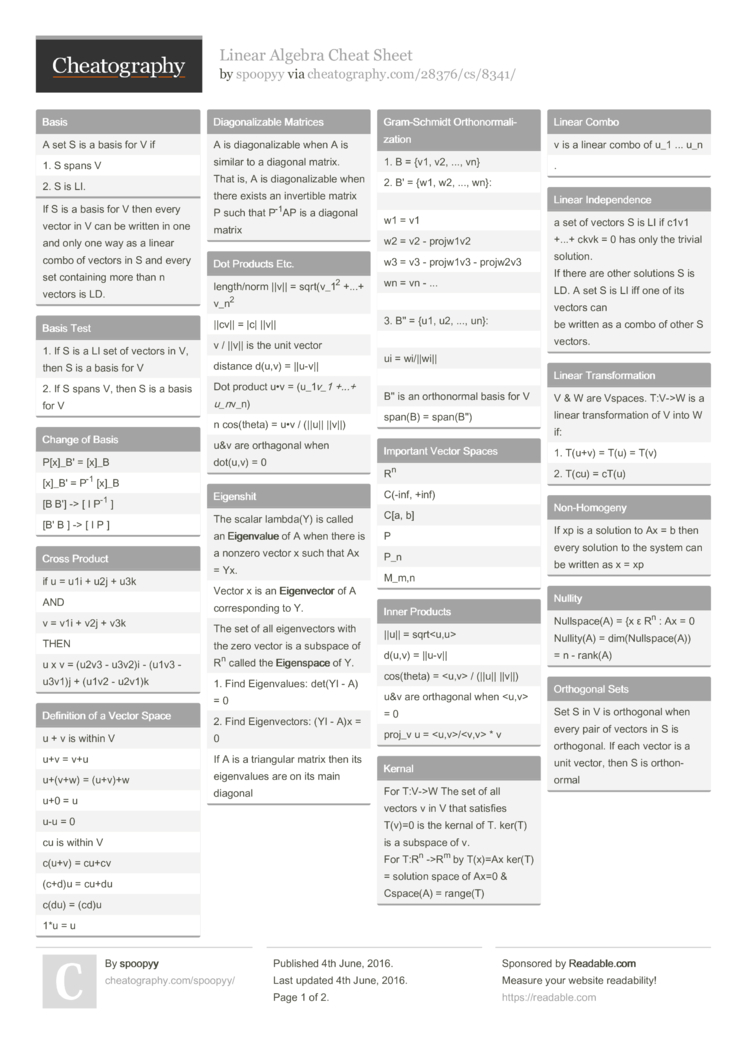Cheatography

# Linear Algebra Cheat Sheet by spoopyy

### Basis

 A set S is a basis for V if 1. S spans V 2. S is LI.
If S is a basis for V then every vector in V can be written in one and only one way as a linear combo of vectors in S and every set containing more than n vectors is LD.

### Basis Test

 1. If S is a LI set of vectors in V, then S is a basis for V 2. If S spans V, then S is a basis for V

### Change of Basis

 P[x]_B' = [x]_B [x]_B' = P-1 [x]_B [B B'] -> [ I P-1 ] [B' B ] -> [ I P ]

### Cross Product

 if u = u1i + u2j + u3k AND v = v1i + v2j + v3k THEN u x v = (u2v3 - u3v2)i - (u1v3 -u3v1)j + (u1v2 - u2v1)k

### Definition of a Vector Space

 u + v is within V u+v = v+u u+(v+w) = (u+v)+w u+0 = u u-u = 0 cu is within V c(u+v) = cu+cv (c+d)u = cu+du c(du) = (cd)u 1*u = u

### Diagon­ali­zable Matrices

 A is diagon­ali­zable when A is similar to a diagonal matrix. That is, A is diagon­ali­zable when there exists an invertible matrix P such that P-1AP is a diagonal matrix

### Dot Products Etc.

 length­/norm ||v|| = sqrt(v­_12 +...+ v_n2 ||cv|| = |c| ||v|| v / ||v|| is the unit vector distance d(u,v) = ||u-v|| Dot product u•v = (u_1v_1 +...+ u_nv_n) n cos(theta) = u•v / (||u|| ||v||) u&v are orthagonal when dot(u,v) = 0

### Eigenshit

 The scalar lambda(Y) is called an Eige­nva­lue of A when there is a nonzero vector x such that Ax = Yx. Vector x is an Eige­nve­ctor of A corres­ponding to Y. The set of all eigenv­ectors with the zero vector is a subspace of Rn called the Eige­nsp­ace of Y. 1. Find Eigenv­alues: det(YI - A) = 0 2. Find Eigenv­ectors: (YI - A)x = 0 If A is a triangular matrix then its eigenv­alues are on its main diagonal

### Gram-S­chmidt Orthon­orm­ali­zation

 1. B = {v1, v2, ..., vn} 2. B' = {w1, w2, ..., wn}: w1 = v1 w2 = v2 - projw1v2 w3 = v3 - projw1v3 - projw2v3 wn = vn - ... 3. B'' = {u1, u2, ..., un}: ui = wi/||wi|| B'' is an orthon­ormal basis for V span(B) = span(B'')

### Important Vector Spaces

 Rn C(-inf, +inf) C[a, b] P P_n M_m,n

### Inner Products

 ||u|| = sqrt d(u,v) = ||u-v|| cos(theta) = / (||u|| ||v||) u&v are orthagonal when = 0 proj_v u = * v

### Kernal

 For T:V->W The set of all vectors v in V that satisfies T(v)=0 is the kernal of T. ker(T) is a subspace of v. For T:Rn ->Rm by T(x)=Ax ker(T) = solution space of Ax=0 & Cspace(A) = range(T)

### Linear Combo

 v is a linear combo of u_1 ... u_n .

### Linear Indepe­ndence

 a set of vectors S is LI if c1v1 +...+ ckvk = 0 has only the trivial solution. If there are other solutions S is LD. A set S is LI iff one of its vectors can be written as a combo of other S vectors.

### Linear Transf­orm­ation

 V & W are Vspaces. T:V->W is a linear transf­orm­ation of V into W if: 1. T(u+v) = T(u) = T(v) 2. T(cu) = cT(u)

### Non-Ho­mogeny

 If xp is a solution to Ax = b then every solution to the system can be written as x = xp

### Nullity

 Nullsp­ace(A) = {x ε Rn : Ax = 0 Nullity(A) = dim(Nu­lls­pac­e(A)) = n - rank(A)

### Orthogonal Sets

 Set S in V is orthogonal when every pair of vectors in S is orthog­onal. If each vector is a unit vector, then S is orthon­ormal

### One-to-One and Onto

 T is one-to-one iff ker(T) = {0} T is onto iff rank(T) = dim(W) If dim(T) = dim(W) then T is one-to-one iff it is onto

### Rank and Nullity of T

 nullity(T) = dim(ke­rnal) rank(T) = dim(range) range(T) + nullity(T) = n (in m_x n) dim(do­main) = dim(range) + dim(ke­rnal)

### Rank of a Matrix

 Rank(A) = dim(Rs­pace) = dim(Cs­pace)

### Similar Matrices

 For square matrices A and A' of order n, A' is similar to A when there exits an invertible matrix P such that A' = P-1 AP

### Spanning Sets

 S = {v1...vk} is a subset of vector space V. S spans V if every vector in v can be written as a linear combo of vectors in S.

### Test for Subspace

 1. u+v are in W 2. cu is in w2 Pages
//media.cheatography.com/storage/thumb/spoopyy_linear-algebra.750.jpg

PDF (recommended)